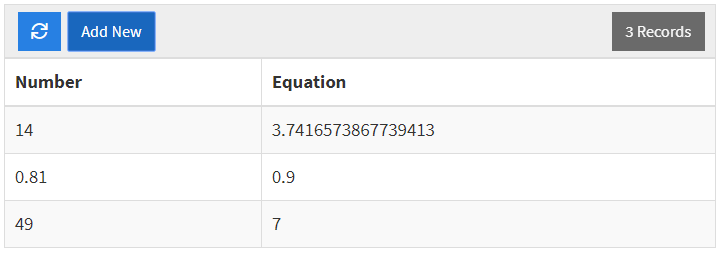This article provides details on our Math Functions that are available for use with the Equation Field.The ABS function generates the absolute value of your field. This function requires one numeric value for input as pictured above. Below is an example of a result of this function in a table.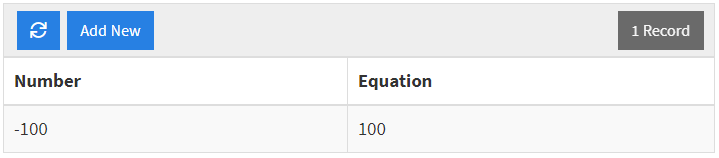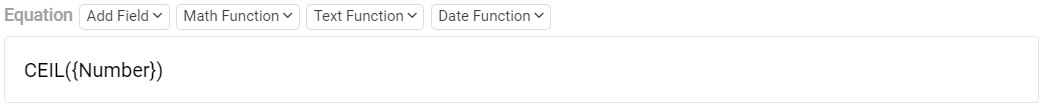The CEIL function generates the ceiling value of your field. The expected input for this function is a single numeric value (as pictured above). Below you can find some sample results of this function.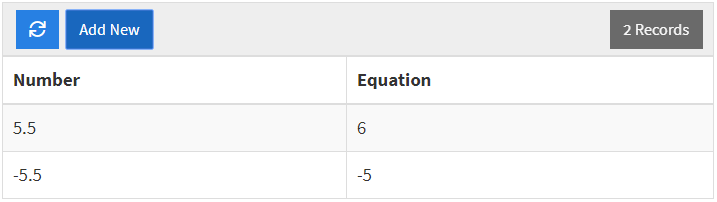The FLOOR function generates the floor value of your field. The expected input for this function is a single numeric value (as pictured above). Below you can find some sample results of this function.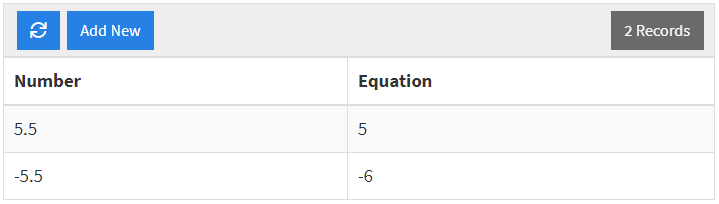ROUND: Use Case 1ROUND: Use Case 2The ROUND function generates a value that is rounded, by default, to the nearest whole number. This function has two different options for input, one takes a single numeric value (ROUND: Use Case 1), and the other takes two (ROUND: Use Case 2). If one numeric value is included, then that value will be rounded to the nearest whole number. If a second numeric value is defined, the first value in the function will be rounded to the nearest nth decimal place.

ROUND: Use Case 1ROUND: Use Case 2 where n = 3ROUND: Use Case 2 where n = -1The SQRT function generates the square root of your fielThe expected input for this function is a single numeric value (as pictured above). Below you can find some sample results of this function.